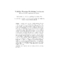## Visibility Planning: Predicting Continuous Period of Unobstructed Views##### Files
CS-TR-4577.ps(456.55 KB)
To perform surveillance tasks effectively, unobstructed views of objects are required e.g. unobstructed video of objects are often needed for gait recognition. As a result, we need to determine intervals for video collection during which a desired object is visible w.r.t. a given sensor. In addition, these intervals are in the future so that the system can effectively plan and schedule sensors for collecting these videos. We describe an approach to determine these visibility intervals. A Kalman filter is first used to predict the trajectories of the objects. The trajectories are converted to polar coordinate representations w.r.t. a given sensor. Trajectories with the same angular displacement w.r.t. the sensor over time can be found by determining intersection points of functions representing these trajectories. Intervals between these intersection points are suitable for video collection. We also address the efficiency issue of finding these intersection points. An obvious brute force approach of $O(N^2)$ exists, where $N$ is the number of objects. This approach suffices when $N$ is small. When $N$ is large, we introduce an optimal segment intersection algorithm of $O(N\log^2N+I)$, $I$ being the number of intersection points. Finally, we model the prediction errors associated with the Kalman filter using a circular object representation. Experimental results that compare the performance of the brute force and the optimal segment intersection algorithms are shown. (UMIACS-TR-2004-22)